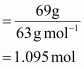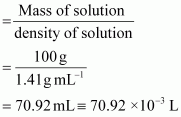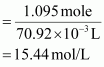Thank you for registering.

One of our academic counsellors will contact you within 1 working day.

Click to Chat

1800-5470-145

+91 7353221155

CART 0

• 0
MY CART (5)

Use Coupon: CART20 and get 20% off on all online Study Material

ITEM
DETAILS
MRP
DISCOUNT
FINAL PRICE
Total Price: Rs.

There are no items in this cart.
Continue Shopping

Calculate the concentration of nitric acid in moles per litre in a sample whichhas a density, 1.41 g mL–1 and the mass per cent of nitric acid in it being 69%.

8 years ago

Mass percent of nitric acid in the sample = 69 % [Given]

Thus, 100 g of nitric acid contains 69 g of nitric acid by mass.

Molar mass of nitric acid (HNO3)

= {1 + 14 + 3(16)} g mol–1

= 1 + 14 + 48

= 63 g mol–1

∴ Number of moles in 69 g of HNO3Volume of 100g of nitric acid solutionConcentration of nitric acid∴Concentration of nitric acid = 15.44 mol/L

8 years ago

given: 1.41 g/mL HNO3 solution
the solution is 69% HNO3

1. calculate the mass of HNO3 in the solution
= 1.41 g/ml x 69%
= 0.9729 g/ml
2. convert the answer in 1 to mole quantity by dividing it with the molar mass of HNO3 which is 63 g/mol
= 0.9729 g/ml divided 63 g/mol
= 0.01544 mol/ml
3. Since molar quantity is expressed as mole per L, you need to multiply the answer in 2 with 1000 (1L = 1000ml)
= 0.01544 mol/ml X 1000
= 15.44 M

The concentration of the solution is 15.44 M.

4 years ago
Molar mass of HNO3 = 63 ,
moles in 69g HNO3 = 69/63 = 1.095
V = 100/1.41 = 70.92ml = 0.07092 L ,
Con. of HNO3 = 1.095/0.07092 = 15.44 M